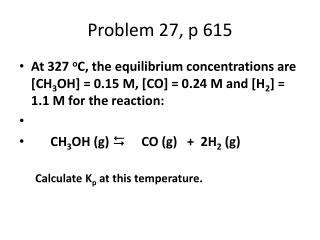DownloadDownload PresentationProblem 27, p 615

# Problem 27, p 615

Download Presentation## Problem 27, p 615

- - - - - - - - - - - - - - - - - - - - - - - - - - - E N D - - - - - - - - - - - - - - - - - - - - - - - - - - -
##### Presentation Transcript

1. Problem 27, p 615 • At 327 oC, the equilibrium concentrations are [CH3OH] = 0.15 M, [CO] = 0.24 M and [H2] = 1.1 M for the reaction: • CH3OH (g) D CO (g) + 2H2 (g) Calculate Kp at this temperature.

2. Problem 31, p. 615 • Consider the following rxn. at a certain temperature: • Fe (s) + 3 O2 (g) D 2 Fe2O3 (s) • An equil. mixture contains 1.0 mole Fe, 0.0010 mole O2 and 2.0 mole Fe2O3 all in a 2.0 L container. Calculate Kc for this reaction.

3. Problem 39, p. 616 • A 1.00 L flask was filled with 2.00 mole gaseous SO2 and 2.00 mole of NO2 and heated After equil. was reached, it was found that 1.30 mole gaseous NO was present. Calculate the value of Kcfor: • SO2 (g) + NO2 (g) D SO3 (g) + NO (g)

4. Problem 52, p. 617 • At a particular temperature, Kc = 4.0 x 10-7 for the reaction: N2O4 (g) D 2NO2 (g) In an expt. 1.0 mole of N2O4 is placed in a 1.00 -L vessel. Calculate the concentrations of N2O4 and NO2 when this reaction reaches equilibrium.

5. Use of Approximation • Approximations can be used if the value of K is so small that the terms (-x) or (+x) will barely have an effect. • Validity of approximation: < 5% • ______[x]______ =<5% [initial concentration]